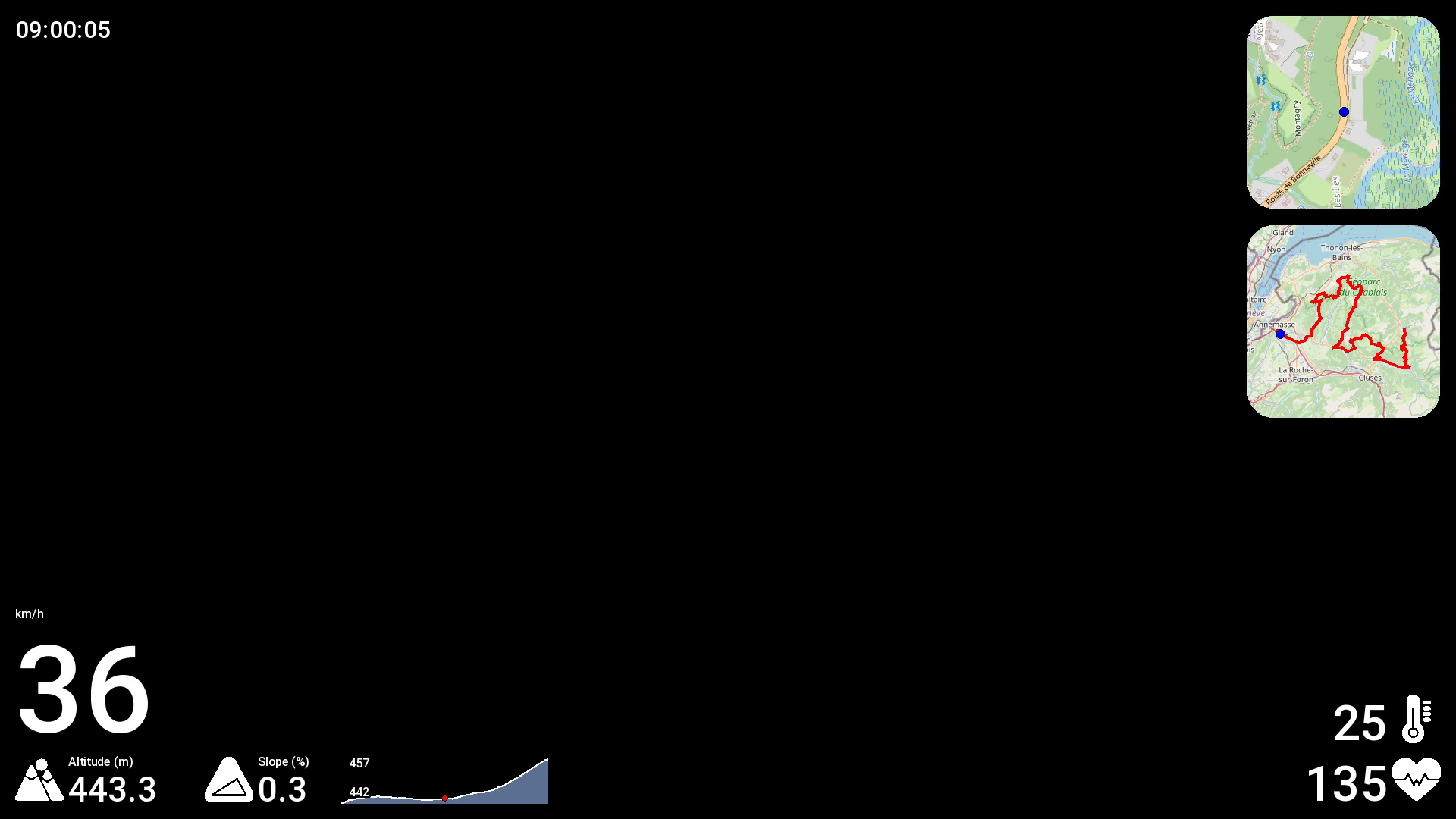# Creating GPX overlay videos on Linux

A tutorial about adding GPX data on top of videos (speed, heart rate, etc)

Category: Linux

Tags: video, linux, gps, gpx, kdenlive

Just because I found this article so good but a bit too long, I'm creating my own.

Up until now, I was relying on the infamous Virb Edit program from Garmin to create videos with GPX data on top. But this software is only available on Mac and Windows, and also outdated and unmaintained, it does not support HEVC videos. So I started looking for another solution that would work on Linux and be ideally dead simple, with no GUI. Also, I was very tired of having to export the GPX data on top of an existing video. Wouldn't it be great to be able to export a video with a transparent background, that I can then put on top of any other footage? GoPro videos, Insta360 videos, any! That would preserve the quality of the video (no need to re-encode it in Virb and then in the final montage software).

I found salvation in gopro-dashboard-overlay! It does all of that! No GUI, can export just a transparent video with the GPX data only but also on top of an existing footage, supports as many formats and codecs as does FFMPEG, and of course works on Linux!

So here is my TL;DR tutorial on how to use it:

# 1. Install the project

``````git clone git@github.com:time4tea/gopro-dashboard-overlay.git
cd gopro-dashboard-overlay
python3 -m venv .env
source .env/bin/activate
pip install gopro-overlay
sudo apt install fonts-roboto
``````

# 2. Setup

``````mkdir ~/.gopro-graphics/
vim ~/.gopro-graphics/ffmpeg-profiles.json
``````

Then:

``````{
"vp9": {
"input": [],
"output": ["-vcodec", "vp9", "-pix_fmt", "yuva420p", "-r", "5"]
},
"mp4": {
"input": [],
"output": ["-vcodec", "libx264", "-r", "25"]
},
"png": {
"input": [],
"output": ["-vcodec", "png"]
}
}
``````

Then:

``````vim ~/Documents/my-layout.xml
``````

Then:

``````<layout>
<composite x="20" y="20" name="date_and_time">
<component type="datetime" x="0" y="0" format="%H:%M:%S" size="32" align="left"/>
<!-- <component type="text" x="0" y="36" size="32">Distance (km): </component>
<component type="metric" x="215" y="36" metric="odo" units="km" size="32" dp="2" /> -->
</composite>

<composite x="20" y="976" name="big_kph">
<!-- 1080 - 20 (margin) - 64 (altitude) - 20 (margin) = y 976 -->
<component type="metric" x="0" y="-160" metric="speed" units="speed" dp="0" size="160" />
<component type="metric_unit" x="0" y="-176" metric="speed" units="speed" size="16">km/h</component>
</composite>

<composite x="20" y="1060" name="altitude">
<component type="icon" x="0" y="-64" file="mountain.png" size="64"/>
<component type="metric_unit" x="70" y="-64" metric="alt" units="alt" size="16">Altitude ({:~C})</component>
<component type="metric" x="70" y="-46" metric="alt" units="alt" dp="1" size="46" />
</composite>

<component type="icon" x="0" y="-64" file="slope-triangle.png" size="64"/>
<component type="text" x="70" y="-64" size="16">Slope (%)</component>
<component type="metric" x="70" y="-46" metric="gradient" dp="1" size="46" />
</composite>

<composite x="1900" y="980" name="temperature">
<component type="icon" x="-64" y="-64" file="thermometer.png" size="64"/>
<component type="metric" x="-70" y="-64" dp="0" size="64" align="right" metric="temp" units="temp"/>
</composite>

<composite x="1900" y="1060" name="heartbeat">
<component type="icon" x="-64" y="-64" file="heartbeat.png" size="64"/>
<component type="metric" x="-70" y="-64" metric="hr" dp="0" size="64" align="right"/>
</composite>

<component type="moving_map" name="moving_map" x="1644" y="20" size="256" zoom="16" corner_radius="35"/>
<component type="journey_map" name="journey_map" x="1644" y="296" size="256" corner_radius="35"/>
</layout>
``````

Then:

``````vim ~/Documents/my-layout-portrait.xml
``````

Then:

``````<layout>
<composite x="1060" y="1816" name="big_kph">
<!-- 1920 - 20 (margin) - 64 (altitude) - 20 (margin) = y 1816 -->
<component type="metric" x="0" y="-160" metric="speed" units="speed" dp="0" size="160" align="right" />
<component type="metric_unit" x="0" y="-192" metric="speed" units="speed" size="32" align="right">km/h</component>
</composite>

<composite x="1060" y="1900" name="heartbeat">
<component type="icon" x="-64" y="-64" file="heartbeat.png" size="64"/>
<component type="metric" x="-70" y="-64" metric="hr" dp="0" size="64" align="right"/>
</composite>
</layout>
``````

# 3. Generate a video

## A transparent video with the data only

``````.env/bin/gopro-dashboard.py --use-gpx-only --gpx ~/Downloads/some-ride.gpx --profile vp9 --layout-xml ~/Documents/my-layout.xml --overlay-size 1920x1080 --units-speed kph --units-altitude meter --units-distance km --units-temperature degC --gps-speed-max 120 --gps-speed-max-units kph ~/Downloads/output.webm
``````

It's going to take some hours. Encoding with VP9 in a .webm container is actually slower than with PNG in .mov container, but the file size is like 100 times smaller. Another way to speed up the processing is to further reduce the final file framerate (30 by default, here in the XML we set it to 5).

That's the kind of result you can expect:A screenshot of the video, in case you can't play it

And the final result, merged with GoPro footage:

All that's left now, is merge this video with an actual footage, sync it, and voilà! I recommend using Kdenlive on Linux..

## The final video right away (footage + overlay)

Make sure the input video has the correct mtime (modified time). Check with `stat file.mp4` or `ls -la file.mp4`. If it's a Insta360 video, and the filename matches `YYYYMMDD_HHMMSS_sss.mp4`, you can update it with this script: https://github.com/rpellerin/dotfiles/blob/master/scripts/updateModifyTimeInsta360File.py

``````.env/bin/gopro-dashboard.py --use-gpx-only --gpx ~/Downloads/some-ride.gpx  --profile mp4 --layout-xml ~/Documents/my-layout-portrait.xml --overlay-size 1080x1920 --units-speed kph --units-altitude meter --units-distance km --units-temperature degC --gps-speed-max 120 --gps-speed-max-units kph --video-time-start file-modified some-video.mp4  ~/Downloads/output.mp4
``````

That's it!